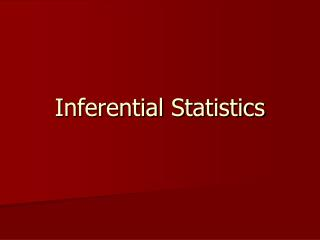# Inferential Statistics - PowerPoint PPT PresentationDownload PresentationInferential Statistics

Inferential Statistics
Download Presentation## Inferential Statistics

- - - - - - - - - - - - - - - - - - - - - - - - - - - E N D - - - - - - - - - - - - - - - - - - - - - - - - - - -
##### Presentation Transcript

1. Inferential Statistics

2. The Logic of Inferential Statistics • Makes inferences about a population from a sample • Assumes a random sample to estimate error • Uses tests of significance which are rooted in the logic of probability sampling

3. Making a Statistical Decision • Step 1: Establishing Type I and Type II Error Risk Levels • Step 2: Selecting the appropriate statistical test • Parametric and Nonparametric Statistics • Statistics of Difference and Relationship • Step 3: Computing the test statistic • Step 4: Consulting the appropriate statistical table • Step 5: Deciding whether or not to reject the null hypothesis.

4. Chi Square • Nonparametric Statistic of difference • Used to identify differences in frequency data. • One Sample Chi Square compares the frequency of attributes of a variable measured at the nominal level. • Chi Square for Contingency Tables compares the frequency of attributes of two or more variables measured at the nominal level.

5. T-test • Parametric statistic of difference • Measures the difference between attributes of an independent variable measured at the nominal level on some dependent variable measured at the interval or ratio level. • The Independent Samples T-test is used when the two groups (independent variable) are independent. • The Paired Samples T-test is used when the two groups (independent variable) are related.

6. Analysis of Variance (ANOVA) • Parametric test of difference • Assesses the extent to which attributes of independent variables measured at the nominal level differ on some dependent variable measured at the interval or ratio level. • A one-way ANOVA is used when there are more than two attributes of a single independent variable measured at the nominal level. • A factorial ANOVA is used when there are more than one independent variables measured at the nominal level.

7. Pearson Product-Moment Correlation • Parametric statistic of relationship • Assess the degree to which two variables measured at the interval or ratio level are linearly related to one another. • A correlation coefficient can range from -1.00 (a perfect negative relationship) to +1.00 (perfect positive relationship) • The coefficient of determination indicates the percentage of variation of one variable that is predicted by knowledge of the other variable.